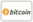Bookcover of Exactly Solvable Q-Extended Nonlinear Classical And Quantum Models
Booktitle:

# Exactly Solvable Q-Extended Nonlinear Classical And Quantum Models

LAP LAMBERT Academic Publishing (2014-10-24 )eligible for voucher
ISBN-13:

### 978-3-659-61969-4

ISBN-10:
3659619698
EAN:
9783659619694
Book language:
English
Blurb/Shorttext:
The quantum or q-calculus is based on finite difference re-scaling and first results in it belong to Euler and Gauss. Developed by Fermat, Jackson, Heine, Jacobi, Ramanujan etc, in XX century it was rediscovered as symmetry of quantum models related to quantum algebras and quantum groups. Noncommutative geometry, fractals, hydrodynamics and quantum theory are fields of its applications.The book is devoted to basic principles of q-caluculs and applications to classical and quantum linear and nonlinear problems. Starting from notion of q-number and q-derivative we study classical and quantum oscillators, heat and Burgers equations. We introduce the concepts of q-analogs of shock solitons, traveling waves and analytic functions, and develop multiple q-calculus to study multi-dimensional problems. By recognizing Fibonacci numbers as q-calculus with golden ratio bases, we construct nonlinear quantum oscillator with Fibonacci spectrum. The book is self contained and pedagogically written as an introduction to the subject for students at advanced undergraduate and graduate level from science and engineering specialties. Also it could be a valuable source for researchers in the field.
Publishing house:
Website:
https://www.lap-publishing.com/
By (author) :
Şengül Nalcı, Oktay K. Pashaev
Number of pages:
272
Published on:
2014-10-24
Stock:
Available
Category:
Theoretical physics
Price:
82.90 €
Keywords:
q-calculus, q-solitons, q-special functions, q-difference equations, q-harmonic oscillator, Fibonacci numbers, Analytic functions, Golden ratio### Categories